9 Breaking the Coulomb barrier by electrostatic acceleration of ions in a device with a fixed inner grid and generating a very high voltageJust some idea´s...   (will not produce net energy)

(9e idea)

Let´s us consider the following:

Fig. 1. Schematic representation of an electrostatic device to generate a very high voltage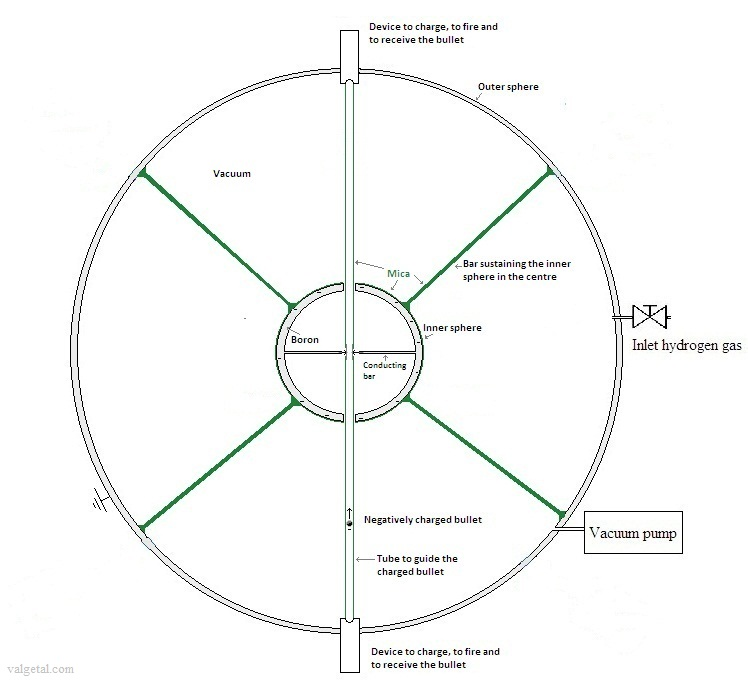Let´s take for the outer diameter of the inner sphere 15 cm and for the outer diameter of the outer sphere 100 cm.

The outer sphere is made of a conducting metal, hollow and strong enough to withhold a vacuum inside. The inside of the outer sphere is pumped vacuum.

The inner sphere is also hollow and is made of a conducting boron compound. Under and above in the inner sphere there is a hole. Along a diameter of the inner sphere there are two thin conducting bars. The inner sphere is kept in the centre by several bars made of mica. The outer surface of the inner sphere is covered by a layer of mica. Mica is a very good electrical insulator and heat resistant.

Under we have a device where we charge a small bullet with a negative charge.  This bullet is "fired" by means of, for example, an electromagnet.  It travels then with a rather high speed towards the opposite side of the outer sphere. On its way it will enter the inner sphere through the hole in the bottom and in the centre of the inner sphere it will touch the two thin metal bars. Because inside a hollow conducting sphere there cannot exists any electric field, the negative charges of the bullet will repel each other and flow through the two thin metal bars to the "outer surface" of the inner sphere  ( the part that is made of the boron compound: the surface just under the mica layer).   This proces is a bit similar as in a Van der Graaff generator. The discharged bullet will then continue its way through the hole in the top of the inner sphere and towards the top of the outer sphere. Here, in a same device as described before, it is charged again with a negative charge and after that fired again, but now downwards. And the same process as before will be repeated.

Which negative voltage we could reach?  -50 MV?  In  wikipedia.org/wiki/Field_electron_emission  it is stated that field emission in pure metals occurs in high electric fields: the gradients are typically higher than 1 gigavolt/meter. But, if nuclear fusion reactions occur (see below), the inner sphere will be heated and the inner sphere will act as a hot cathode and will probably emit electrons and loose its negative charge (see also: wikipedia.org/Thermionic_emission This would be quite a disadvantage...  This is the reason that the inner sphere has been covered by a layer of mican: this material is a very good insulator and hopefully it will keep the electrons in the inner sphere.

In air electrical discharge will occur with 3 MV/m (found in internet). But the space around the inner sphere is vacuum, so this will not take place.

The dielectric breakdown for mica takes place with 160 MV/m ( see physics.info/dielectrics/ ).

The distance between the outer and the inner sphere is in the above configuration about 42,5 cm, so the maximum allowed voltage is 160x0,425 = 68 MV.  If the potential difference between inner and outer (earthed) sphere is no more then - 50 MV, the mica bars should therefore be able to keep the inner sphere in the centre and to insulate its negative electrical charge.

---

Let´s suppose that we have managed to charge the inner sphere negatively with a very high voltage of  -50 MV.

Now we let in some hydrogen. Because of the very strong electric field it will be ionized. The positive hydrogen ions will be accelerated towards the negatively charged inner sphere.

With a voltage of - 50 MV, the ions will obtain an energy of 50 MeV.

Could it be possible perhaps that this would cause a fusion reaction when the hydrogen ion collide with the boron material of the inner sphere?

---

Let´s consider the boron-hydrogen fusion reaction:

115B + 11H  -> 3 42He  + 8,68 MeV   (see en.wikipedia.org/wiki/Aneutronic_fusion)

No noxious neutrons are produced and the 3 positive  42He+ particles will move with high speed out of the centre increasing the voltage. (They could perhaps even be used to generate directly electricity.)

The strong nuclear force works until ± 2 fm = 2.10 -15 m  (see: wiki/Nuclear_force )

Z B  = 5  (amount of positive protons in a boron ion)

Z 1    = 1  (only one positive proton in a hydrogen ion)
k is the Coulomb's constant = 9,0×109 N m² C−2
1 eV = 1,6 . 10
-19  J
= >
Ucoul =  k . Z B . Z 1 . e 2  / r  =  9.0 . 10 9 . 5 . 1 . (1,6.10
-19 ) 2  / (2.10 -15)  = 0,6.10 -12  Nm =    0,6 .10 -12   J  =  3,6. MeV

(see: wiki/Coulomb_barrier )

So to break the coulomb barrier between a boron nucleus and hydrogen nucleus about 4  MeV energy is needed (note: according this very simple calculation).  If a hydrogen ion is accelerated from about the outer sphere to the surface of the inner sphere and achieves a kinetic energy of 50 MeV, this should be more than enough to cause a nuclear fusion reaction.  (If it would collide with a right angle with a boron nucleus...)

The nuclear fusion reactions will generate heat, which could be used to warm up water outside the outer sphere.

The inlet of hydrogen can be controlled, so the generating of heat as well.

---

Calculation of the charge of the inner sphere: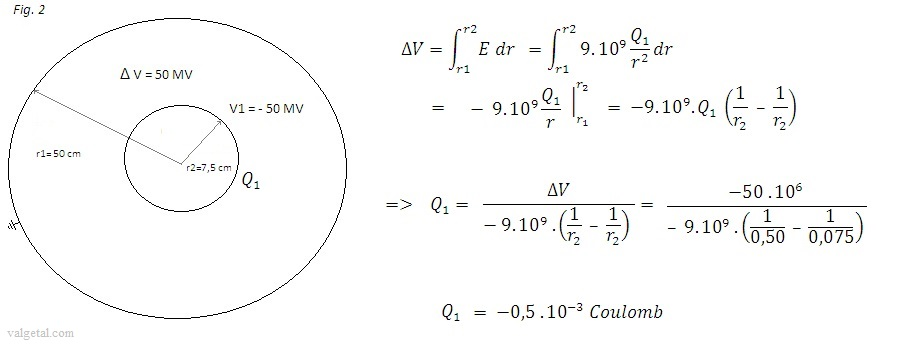Calculation of the electrical energy of the inner sphere:

If the inner sphere would be alone in space, its energy would be:

Energy = ½ Q.V = ½ . -0,5.10-3 . 50.10= 12500 J   (Fundamentele natuurkunde, page 173, Marcelo Alonso & Edward J. Finn & Natuurkunde op Corspusculaire grondslag Deel 4V blz.45, and also see http://en.wikipedia.org/wiki/Electrostatic_potential_energy

)

1 ltr diesel = about 40 MJ,  so the charged inner sphere will contain an energy equivalent to 0,3 gram of diesel.  This is not too much....

Calculations on the bullet:

Suppose we fire every second a bullet and we charge the bullet negatively with 1200 V.

If the bullet would be alone in space we get: V = 9.109.Q/r   (in reality the bullet is surrounded by other material, but I suspect that more or less we will get an idea of the charge of the bullet with this formula)

Let´s take for rbullet=0,05 m , then  Qbullet = -1200.0,05/(9.109) = - 6,7.10-9 Coulomb

Let´s suppose that the bullet discharges all charge inside the inner sphere.
To reach a voltage difference of - 50 MV with Q1= -0,5.10-3 Coulomb, we need to fire the bullet
0,5.10-3 / ( 6,7.
10-9) = 74627 times (seconds) = 74627 /(24.60.60) = 0.86 day.

In one day we could charge the inner sphere to a voltage of - 50 MV, according this simple calculations (One day isn´t that long..)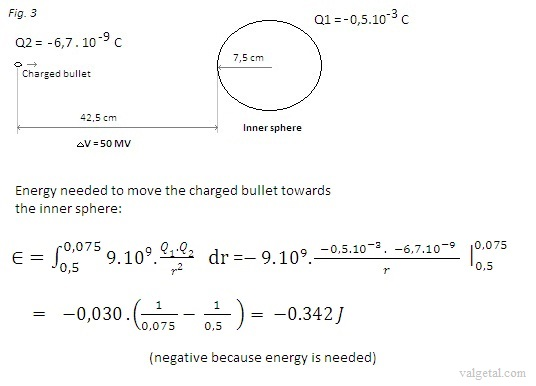To charge the inner sphere we need to fire the bullet every second during more or less one day:

Energy to charge the inner sphere < 0,342 . 24.60.60 . 0.86  = 254112 J (equivalent to about 0,6 gram diesel)

In reality this energy will be is less, because in the beginning the inner sphere is not fully charged.

---

Dielectric breakdown:

Electric field just outside the inner sphere: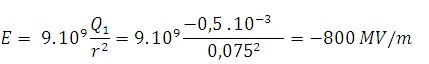This is a lot higher than the dielectric breakdown for mica ( = 160 MV/m)....

---

In this paper the breakdown field strength and resistivity of sputter-deposited Al2O3, SiO2 and Si3N4 layers are investigated in the temperature range between room temperature and 400 °C. All the investigated layers showed excellent insulation properties, even at elevated sample temperature, see:

Here we read that such a layer of Al2O3 had a breakdown field strength of 6,20 MV/cm = 620 MV/m  and SiO2  560MV/m.

See also: wikipedia.org/wiki/Dielectric_strength Here we read that diamond has a breakdown field strength of 2000 MV/m. Would be a little bit expensive..

###### Mylar (® is DuPont's brand name for polyester film) has a breakdown field strength of  7500 Volt/mil  (a mil is 1/1000  inch ) = 295 V/m so better than Mica.

---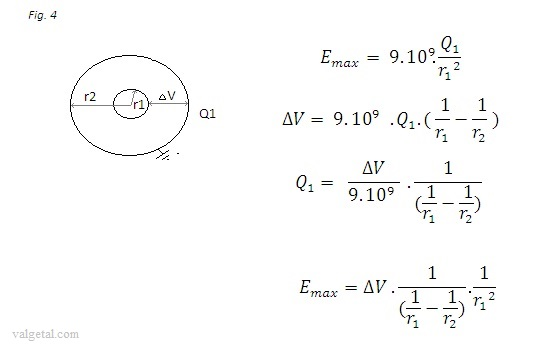With above formulas we calculated the following:

 r1 r2 ΔV Q1 Emax 10 cm 30 cm 50 MV 0,83.10-3 C 750 MV/m 4 cm 50  cm 50 MV 1359 MV/m 7,5 cm 50 cm 50 MV 17.10-3 C 785 MV/m 150 cm 200 cm 50 MV 133 MV/m 10 cm 20 cm 50 MV 1000 MV/m

So the larger the dimensions, the smaller is the maximum electrical field strength, but also the more difficult to pump vacuum and to charge the inner sphere.

---

The charge of the inner sphere is: 0,5.10-3-> divided by 1,6.10-19  C => 3,125.1015  proton charges
After colliding 3,125.1015  protons on the inner sphere its charge (voltage ) would be zero.
If all these protons would cause fusion, the energy generated would be:
Ɛ = 3,125.1015 . 8,68 MeV = 2,7 . 1016 MeV = 4340 J
The electrical energy of the loaded inner sphere is: 12500 J

Conclusion: perhaps it would be possible to generate nuclear fusion reactions, but with 50 MV voltage difference
it´s impossible to generate net energy.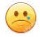Questions/doubts:

The mica layer will catch a part of the incoming hydrogen ions. And not all the ions that come through it will cause a fusion reaction. So what will be the efficiency? I suspect this will be a problem...

You only gain 8,68 MeV and the proton colliding had an energy of 50 MeV.... but the inner sphere should in theory maintain its negative voltage, or even increase it because it looses three 42He+ particles. It would be like throwing stones in a hole: the hole will not become shallower, so you can keep throwing stones in it.. But what is the energy of the 42He+ particles?  They get their energy from the 8,68 MeV. To make the whole process effective, the voltage must be lower then 8,68 MV... But if you apply for example only 1 MV, how many of the incoming protons will cause a fusion reaction?

Part of the 50 MeV of an incoming proton will be converted into heat. If we could regain this heat, but a bit difficult I suspect...

To avoid electrical discharging of the inner sphere by field emission, the layer of mica on the surface of the inner sphere should be as thick as possible. But to avoid that too many incoming hydrogen ions are caught by the mica this layer should be as thin as possible. The right thickness should be determined by experiment.

Perhaps we could make the inner sphere in the following way: outside a layer of borron, to catch the incoming protons for the fusion reactions. Then a layer of insulating material (mica, mylar, diamant?). Then some conducting materiaal to build up a negative electrical charge. The outside layer will probable loose some electron by electron emision, but this will stop once due to the limited amount of free electrons. The insulating layer will hopefully prevent that the negative charge in the inside conducting material is lost.

The device to charge, fire and receive the bullet.. how wil this be?

Will the bullet really discharge when it touches the thin metal bars? Perhaps its speed will be too high..20 February 2014      by  Rinze Joustra        www.valgetal.com